# numpy.cov¶

numpy.cov(m, y=None, rowvar=True, bias=False, ddof=None, fweights=None, aweights=None)[source]

Estimate a covariance matrix, given data and weights.

Covariance indicates the level to which two variables vary together. If we examine N-dimensional samples,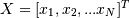, then the covariance matrix element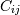is the covariance of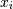and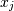. The element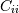is the variance of.

See the notes for an outline of the algorithm.

Parameters: m : array_like A 1-D or 2-D array containing multiple variables and observations. Each row of m represents a variable, and each column a single observation of all those variables. Also see rowvar below. y : array_like, optional An additional set of variables and observations. y has the same form as that of m. rowvar : bool, optional If rowvar is True (default), then each row represents a variable, with observations in the columns. Otherwise, the relationship is transposed: each column represents a variable, while the rows contain observations. bias : bool, optional Default normalization (False) is by (N - 1), where N is the number of observations given (unbiased estimate). If bias is True, then normalization is by N. These values can be overridden by using the keyword ddof in numpy versions >= 1.5. ddof : int, optional If not None the default value implied by bias is overridden. Note that ddof=1 will return the unbiased estimate, even if both fweights and aweights are specified, and ddof=0 will return the simple average. See the notes for the details. The default value is None. New in version 1.5. fweights : array_like, int, optional 1-D array of integer freguency weights; the number of times each observation vector should be repeated. New in version 1.10. aweights : array_like, optional 1-D array of observation vector weights. These relative weights are typically large for observations considered “important” and smaller for observations considered less “important”. If ddof=0 the array of weights can be used to assign probabilities to observation vectors. New in version 1.10. out : ndarray The covariance matrix of the variables.

corrcoef
Normalized covariance matrix

Notes

Assume that the observations are in the columns of the observation array m and let f = fweights and a = aweights for brevity. The steps to compute the weighted covariance are as follows:

```>>> w = f * a
>>> v1 = np.sum(w)
>>> v2 = np.sum(w * a)
>>> m -= np.sum(m * w, axis=1, keepdims=True) / v1
>>> cov = np.dot(m * w, m.T) * v1 / (v1**2 - ddof * v2)
```

Note that when a == 1, the normalization factor v1 / (v1**2 - ddof * v2) goes over to 1 / (np.sum(f) - ddof) as it should.

Examples

Consider two variables,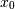and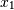, which correlate perfectly, but in opposite directions:

```>>> x = np.array([[0, 2], [1, 1], [2, 0]]).T
>>> x
array([[0, 1, 2],
[2, 1, 0]])
```

Note howincreases whiledecreases. The covariance matrix shows this clearly:

```>>> np.cov(x)
array([[ 1., -1.],
[-1.,  1.]])
```

Note that element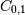, which shows the correlation betweenand, is negative.

Further, note how x and y are combined:

```>>> x = [-2.1, -1,  4.3]
>>> y = [3,  1.1,  0.12]
>>> X = np.vstack((x,y))
>>> print(np.cov(X))
[[ 11.71        -4.286     ]
[ -4.286        2.14413333]]
>>> print(np.cov(x, y))
[[ 11.71        -4.286     ]
[ -4.286        2.14413333]]
>>> print(np.cov(x))
11.71
```

numpy.correlate

numpy.histogram